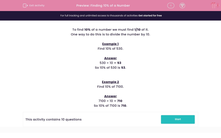# Finding 10% of a Number

In this worksheet, students must find 10% of the given numbers.Key stage:  KS 2

Curriculum topic:   Maths and Numerical Reasoning

Curriculum subtopic:   Percentages

Difficulty level:#### Worksheet Overview

To find 10% of a number we must find 1/10 of it.

One way to do this is to divide the number by 10.

Example 1

Find 10% of 530.

530 ÷ 10 = 53

So 10% of 530 is 53.

Example 2

Find 10% of 7100.